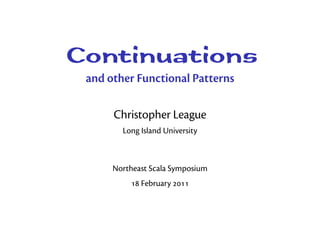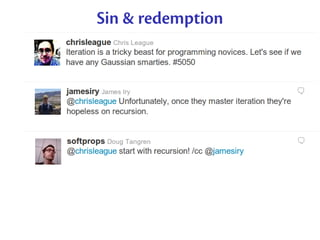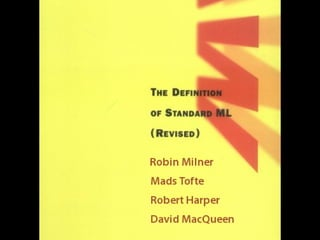Successfully reported this slideshow.

# Scala Functional Patterns

5

Share×
1 of 33
1 of 33

# Scala Functional Patterns

5

Share

Continuations and other Functional Patterns. Northeast Scala Symposium, 18 Februar 2011.

Continuations and other Functional Patterns. Northeast Scala Symposium, 18 Februar 2011.

## More Related Content

### Related Books

Free with a 14 day trial from Scribd

See all

### Related Audiobooks

Free with a 14 day trial from Scribd

See all

### Scala Functional Patterns

1. 1. Continuations and other Functional Patterns Christopher League Long Island University Northeast Scala Symposium  February 
2. 2. Sin & redemption
3. 3. Java classes → SML runtime Standard ML of New Jersey v110.30 [JFLINT 1.2] - Java.classPath := [”/home/league/r/java/tests”]; val it = () : unit - val main = Java.run ”Hello”; [parsing Hello] [parsing java/lang/Object] [compiling java/lang/Object] [compiling Hello] [initializing java/lang/Object] [initializing Hello] val main = fn : string list -> unit - main [”World”]; Hello, World val it = () : unit - main []; uncaught exception ArrayIndexOutOfBounds raised at: Hello.main([Ljava/lang/String;)V - ˆD
4. 4. OO runtime ← functional languages Scala Clojure F etc.
5. 5. Patterns . Continuation-passing style . Format combinators . Nested data types eme Higher-order {functions, types}
6. 6. Pattern : Continuations A continuation is an argument that represents the rest of the computation meant to occur after the current function.
7. 7. Explicit continuations – straight-line def greeting [A] (name: String) (k: => A): A = printk(”Hello, ”) { printk(name) { printk(”!n”)(k) }} def printk [A] (s: String) (k: => A): A = { Console.print(s); k } scala> greeting(”Scala peeps”) { true } Hello, Scala peeps! res0: Boolean = true
8. 8. Pay it forward... Current function can ‘return’ a value by passing it as a parameter to its continuation.
9. 9. Explicit continuations – return values def plus [A] (x: Int, y: Int) (k: Int => A): A = k(x+y) def times [A] (x: Int, y: Int) (k: Int => A): A = k(x*y) def less[A] (x: Int, y: Int) (kt: =>A) (kf: =>A):A = if(x < y) kt else kf def test[A](k: String => A): A = plus(3,2) { a => times(3,2) { b => less(a,b) {k(”yes”)} {k(”no”)} }} scala> test{printk(_){}} yes
10. 10. Delimited continuations reset Serves as delimiter for CPS transformation. shift Captures current continuation as a function (up to dynamically-enclosing reset) then runs speciﬁed block instead.
11. 11. Delimited continuations def doSomething0 = reset { println(”Ready?”) val result = 1 + ˜˜˜˜˜˜˜˜ * 3 println(result) } ink of the rest of the computation as a function with the hole as its parameter.
12. 12. Delimited continuations def doSomething1 = reset { println(”Ready?”) val result = 1 + special * 3 println(result) } def special = shift { k: (Int => Unit) => println(99); ”Gotcha!” } shift captures continuation as k and then determines its own future.
13. 13. Delimited continuations def doSomething1 = reset { println(”Ready?”) val result = 1 + special * 3 println(result) } def special = shift { k: (Int => Unit) => println(99); ”Gotcha!” } scala> doSomething1 Ready? 99 res0: java.lang.String = Gotcha!
14. 14. Continuation-based user interaction def interact = reset { val a = ask(”Please give me a number”) val b = ask(”Please enter another number”) printf(”The sum of your numbers is: %dn”, a+b) } scala> interact Please give me a number answer using: submit(0xa9db9535, ...) scala> submit(0xa9db9535, 14) Please enter another number answer using: submit(0xbd1b3eb0, ...) scala> submit(0xbd1b3eb0, 28) The sum of your numbers is: 42
15. 15. Continuation-based user interaction val sessions = new HashMap[UUID, Int=>Unit] def ask(prompt: String): Int @cps[Unit] = shift { k: (Int => Unit) => { val id = uuidGen printf(”%snanswer using: submit(0x%x, ...)n”, prompt, id) sessions += id -> k }} def submit(id: UUID, data: Int) = sessions(id)(data) def interact = reset { val a = ask(”Please give me a number”) val b = ask(”Please enter another number”) printf(”The sum of your numbers is: %dn”, a+b) }
16. 16. Pattern : Format combinators [Danvy ] Typeful programmers covet printf. int a = 5; int b = 2; float c = a / (float) b; printf(”%d over %d is %.2fn”, a, b, c); Cannot type-check because format is just a string. What if it has structure, like abstract syntax tree?
17. 17. Typed format speciﬁers val frac: Int => Int => Float => String = d & ” over ” & d & ” is ” & f(2) & endl | val grade: Any => Double => Unit = ”Hello, ”&s&”: your exam score is ”&pct&endl |> val hex: (Int, Int, Int) => String = uncurried(”#”&x&x&x|) (Type annotations are for reference – not required.) scala> println(uncurried(frac)(a,b,c)) 5 over 2 is 2.50 scala> grade(”Joshua”)(0.97) Hello, Joshua: your exam score is 97% scala> println(”Roses are ”&s | hex(250, 21, 42)) Roses are #fa152a
18. 18. Buﬀer representation type Buf = List[String] def put(b: Buf, e: String): Buf = e :: b def finish(b: Buf): String = b.reverse.mkString def initial: Buf = Nil
19. 19. Operational semantics def lit(m:String)(k:Buf=>A)(b:Buf) = k(put(b,m)) def x(k:Buf=>A)(b:Buf)(i:Int) = k(put(b,i.toHexString)) def s(k:Buf=>A)(b:Buf)(o:Any) = k(put(b,o.toString)) (Not the actual implementation.) lit(”L”)(finish)(initial) ”L” x(finish)(initial)(42) ”2a” where: type Buf = List[String] def put(b: Buf, e: String): Buf = e :: b def finish(b: Buf): String = b.reverse.mkString def initial: Buf = Nil
20. 20. Function composition (lit(”L”) & x) (finish) (initial) (2815) lit(”L”)(x(finish)) (initial) (2815) lit(”L”)(λ b0.λ i.finish(i.toHex :: b0)) (initial) (2815) (λ b1.λ i.finish(i.toHex :: ”L” :: b1)) (initial) (2815) finish(2815.toHex :: ”L” :: initial) List(”aff”,”L”).reverse.mkString ”Laff” where: def lit(m:String)(k:Buf=>A)(b:Buf) = k(put(b,m)) def x(k:Buf=>A)(b:Buf)(i:Int) = k(put(b,i.toHexString)) def s(k:Buf=>A)(b:Buf)(o:Any) = k(put(b,o.toString))
21. 21. Combinator polymorphism What is the answer type? x: ∀A.(Buf => A) => Buf => Int => A finish: Buf => String x(x(x(finish))) A ≡ String A ≡ Int => String A ≡ Int => Int => String
22. 22. Type constructor polymorphism trait Compose[F[_],G[_]] { type T[X] = F[G[X]] } trait Fragment[F[_]] { def apply[A](k: Buf=>A): Buf=>F[A] def & [G[_]] (g: Fragment[G]) = new Fragment[Compose[F,G]#T] { def apply[A](k: Buf=>A) = Fragment.this(g(k)) } def | : F[String] = apply(finish(_))(initial) }
23. 23. Combinator implementations type Id[A] = A implicit def lit(s:String) = new Fragment[Id] { def apply[A](k: Cont[A]) = (b:Buf) => k(put(b,s)) } type IntF[A] = Int => A val x = new Fragment[IntF] { def apply[A](k: Cont[A]) = (b:Buf) => (i:Int) => k(put(b,i.toHexString)) }
24. 24. Pattern : Nested data types Usually, a polymorphic recursive data type is instantiated uniformly throughout: trait List[A] case class Nil[A]() extends List[A] case class Cons[A](hd:A, tl:List[A]) extends List[A] What if type parameter of recursive invocation diﬀers?
25. 25. Weird examples trait Weird[A] case class Wil[A]() extends Weird[A] case class Wons[A](hd: A, tl: Weird[(A,A)]) extends Weird[A] // tail of Weird[A] is Weird[(A,A)] val z: Weird[Int] = Wons(1, Wil[I2]()) val y: Weird[Int] = Wons(1, Wons((2,3), Wil[I4])) val x: Weird[Int] = Wons( 1, Wons( (2,3), Wons( ((4,5),(6,7)), Wil[I8]()))) type I2 = (Int,Int) type I4 = (I2,I2) type I8 = (I4,I4)
26. 26. Square matrices [Okasaki ] Vector[Vector[A]] is a two-dimensional matrix of elements of type A. But lengths of rows (inner vectors) could diﬀer. Using nested data types, recursively build a type constructor V[_] to represent a sequence of a ﬁxed number of elements. en, Vector[V[A]] is a well-formed matrix, and V[V[A]] is square.
27. 27. Square matrix example scala> val m = tabulate(6){(i,j) => (i+1)*(j+1)} m: FastExpSquareMatrix.M[Int] = Even Odd Odd Zero (((),((((),(1,2)),((3,4),(5,6)) ),(((),(2,4)),((6,8),(10,12))))),(((((),(3,6)),(( 9,12),(15,18))),(((),(4,8)),((12,16),(20,24)))),( (((),(5,10)),((15,20),(25,30))),(((),(6,12)),((18 ,24),(30,36)))))) scala> val q = m(4,2) q: Int = 15 scala> val m2 = m updated (4,2,999) m2: FastExpSquareMatrix.M[Int] = Even Odd Odd Zero (((),((((),(1,2)),((3,4),(5,6)) ),(((),(2,4)),((6,8),(10,12))))),(((((),(3,6)),(( 9,12),(15,18))),(((),(4,8)),((12,16),(20,24)))),( (((),(5,10)),((999,20),(25,30))),(((),(6,12)),(( 18,24),(30,36))))))
28. 28. Analogy with fast exponentiation fastexp r b 0 = r fastexp r b n = fastexp r (b2 ) n/2 if n even fastexp r b n = fastexp (r · b) (b2 ) n/2 otherwise For example: fastexp 1 b 6 = Even fastexp 1 (b2 ) 3 = Odd 2 fastexp (1 · b2 ) (b2 ) 1 = Odd 2 22 fastexp (1 · b2 · b2 ) (b2 ) 0 = Zero 2 1 · b2 · b2
29. 29. Fast exponentiation of product types type U = Unit type I = Int fastExp U I 6 = // Even fastExp U (I,I) 3 = // Odd fastExp (U,(I,I)) ((I,I),(I,I)) 1 = // Odd fastExp ((U,(I,I)),((I,I),(I,I))) // Zero (((I,I),(I,I)),((I,I),(I,I))) 0 = ((U,(I,I)),((I,I),(I,I)))
30. 30. Implementation as nested data type trait Pr[V[_], W[_]] { type T[A] = (V[A],W[A]) } trait M [V[_],W[_],A] case class Zero [V[_],W[_],A] (data: V[V[A]]) extends M[V,W,A] case class Even [V[_],W[_],A] ( next: M[V, Pr[W,W]#T, A] ) extends M[V,W,A] case class Odd [V[_],W[_],A] ( next: M[Pr[V,W]#T, Pr[W,W]#T, A] ) extends M[V,W,A] type Empty[A] = Unit type Id[A] = A type Matrix[A] = M[Empty,Id,A]
31. 31. anks! league@contrapunctus.net @chrisleague github.com/league/ slidesha.re/eREMXZ scala-fun-patterns Danvy, Olivier. “Functional Unparsing” J. Functional Programming (), . Okasaki, Chris. “From Fast Exponentiation to Square Matrices: An Adventure in Types” Int’l Conf. Functional Programming, .